# Impulse Response Of Second Order Linear System Notes - Mechanical Engineering

## Mechanical Engineering: Impulse Response Of Second Order Linear System Notes - Mechanical Engineering

The document Impulse Response Of Second Order Linear System Notes - Mechanical Engineering is a part of Mechanical Engineering category.
All you need of Mechanical Engineering at this link: Mechanical Engineering

Impulse Response of Second-Order Linear System

Suppose now that we consider the general motion of the system in Fig. 2–1, i.e., we consider motion to a general force F(t). Then, recalling the result from earlier, the differential equation of motion is given as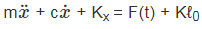(2–8)

It is noted that the equilibrium point of the system in Eq. (2–8) is xeq = ℓ0, we can define the variable y = x − xeq and rewrite Eq. (2–8) in terms of y to give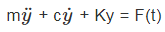(2–9)

Now suppose that F(t) is the following function: F(t) =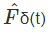(2–10)

where δ(t) is defined as follows: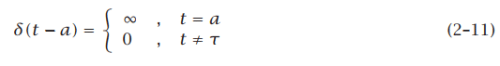The function δ(t) is called the Dirac delta function or the unit impulse function. It is known that the Dirac delta function satisfies the following properties: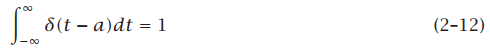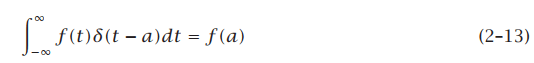where f (t) is an arbitrary function. For simplicity, consider the case where Fˆ = 1, i.e., the case of unit impulse being applied to the system. Also, let g(t) be the response to the input δ(t), i.e., consider the system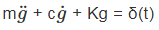(2–14)

Let T be a value of t such that T > 0. Then, integrating Eq. (2–14) from zero to T, we have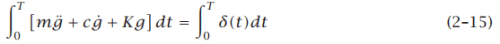Now we have the following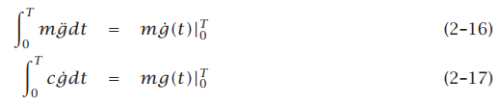Taking the limit as T → 0 from above, we obtain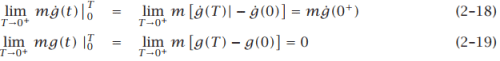Furthermore, because the position of the mass cannot change during the application of an instantaneous impulse, we see that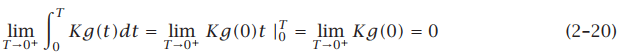Using the results of Eqs. (2–18), (2–19) and (2–20) in Eq. (2–15), we obtain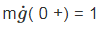(2–21)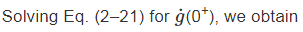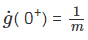(2–22)

It is seen that, for the case where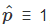, the results of Eq. (2–7) and Eq. (2–22) are identical. More specifically, as we saw above, the effect of a unit impulsive force on a resting particle of mass m is to provide an initial velocity of magnitude 1/m while the response of a second-order linear system to a unit impulse function (i.e., the Dirac delta function) is to provide an initial velocity of magnitude 1/m. Consequently, the physics of an impulsive force on a resting particle is identical to the mathematics of the impulse response of the system to a unit impulse.
Now that we know that the response of a second-order resting system is to change the velocity (while leaving position unchanged), we can use this fact to obtain the impulse response g(t). In particular, assuming an underdamped system, we know that the general form of the free response is given as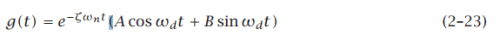where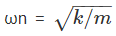is the natural frequency, ζ is the damping ratio, and ωd = ωn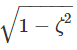is the damped natural frequency. Differentiating this last equation, we have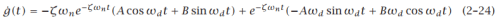Noting that g(0) = 0 and that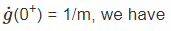A = 0                                                                                                                 (2–25)
B =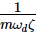(2–26)

Therefore, the response of the system to a unit impulse at t = 0 is given as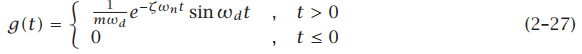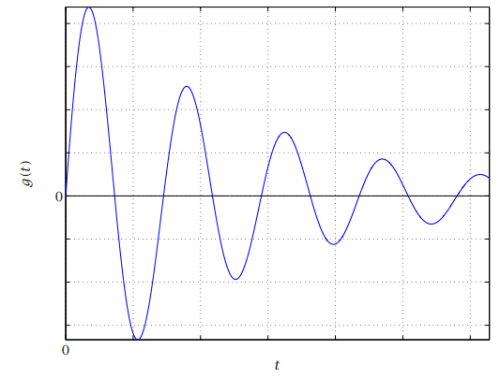Figure 2–2 Schematic of Impulse Response of Underdamped Second-Order Linear System

The document Impulse Response Of Second Order Linear System Notes - Mechanical Engineering is a part of Mechanical Engineering category.
All you need of Mechanical Engineering at this link: Mechanical EngineeringUse Code STAYHOME200 and get INR 200 additional OFF

Track your progress, build streaks, highlight & save important lessons and more!

,

,

,

,

,

,

,

,

,

,

,

,

,

,

,

,

,

,

,

,

,

;